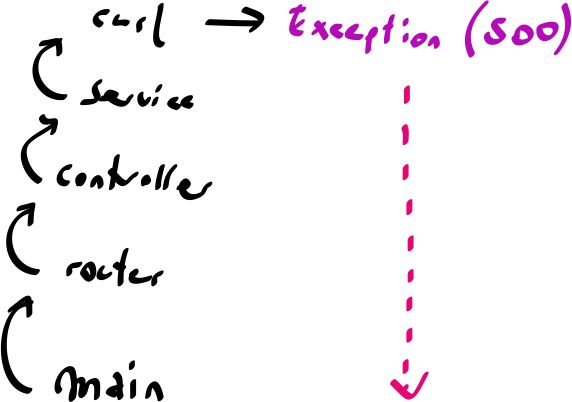# Overview for 'igor'

## Probabilistic AutomataWritten by igorw / Original link on Aug. 1, 2020Probabilistic Automata Have you seen those twitter bots that generate fake or parody tweets? The ones that look almost legit but are pretty much complete jibberish when you read them? Quite often, such accounts use a so-called markov chain to generate the text. A markov chain is a state machine tha…

## Stack Machines: CompilersWritten by igorw / Original link on Aug. 24, 2019

Stack Machines: Compilers fundamentals << rpn-calculator << shunting-yard << io << jumps << conditionals << comments << calls << variables << stack-frames << heap << compilers This is a guest post by the one and only Anthony Ferrara…

## Stack Machines: Stack FramesWritten by igorw / Original link on Aug. 24, 2019Stack Machines: Stack Frames fundamentals << rpn-calculator << shunting-yard << io << jumps << conditionals << comments << calls << variables << stack-frames << heap << compilers The last two posts introduced two separate concepts:…